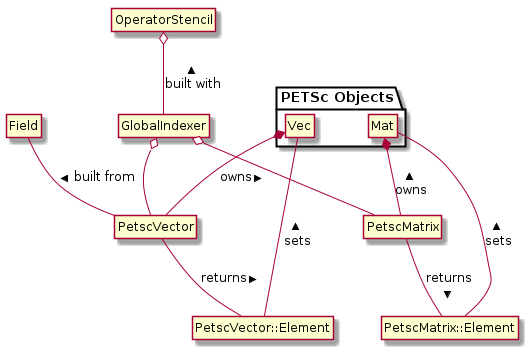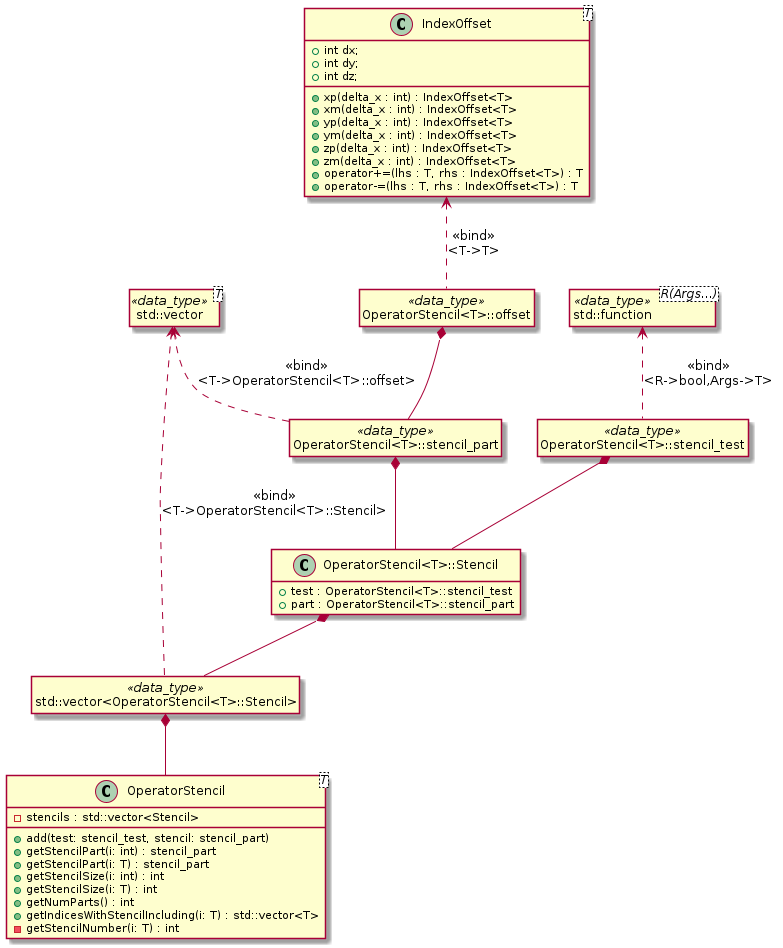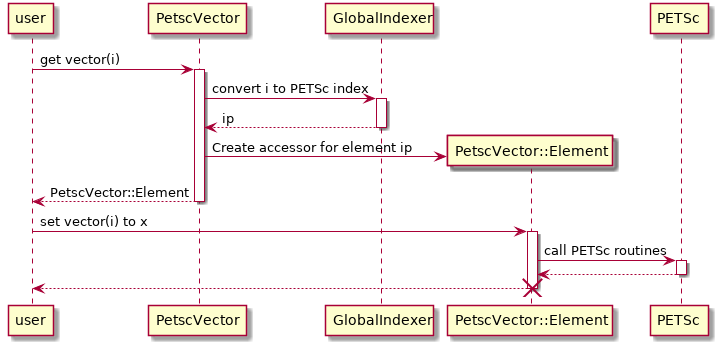# PETSc interface#

The Portable, Extensible Toolkit for Scientific Computation (PETSc) provides a large collection of numerical routines and solvers. It is used in BOUT++ for Time integration and Laplacian inversion. However, it provides quite a low-level C interface which is often difficult to use and bears little resemblance to the data model of BOUT++. This is particularly the case when making use of the Mat and Vec data-types for linear solvers (such as the Laplacian inversions). Doing so requires the developer to:

1. Flatten a Field into a 1-D PETSc Vec object.

• Must decide which guard cells to include in Vec

• Must convert between BOUT++ indices (local) and indices used in PETSc Vec (global)

2. Use a PETSc Mat object to represent a finite-difference operator.

• Again, must convert between local and global indices

• Must determine sparsity pattern of matrix

• If taking field-aligned derivatives, must perform interpolation

3. Call a Krylov solver with a preconditioner.

4. Convert the resulting Vec object back into a Field.

These tasks are error-prone and have historically been re-implemented each time a PETSc solver is to be used. An interface to PETSc has now been added which greatly simplifies its use. Furthermore, it has been thoroughly unit-tested which should ensure improved reliability.

## Overall Structure#Fig. 24 A UML diagram showing the design of the PETSc interface and the relationships between different components.#

Based on the finite difference operator being inverted, a user constructs an OperatorStencil object which is used to describe the interdependencies between cells in the grid. This is used when constructing a GlobalIndexer to work out which cells should be included in a Vec object. If needed, it will also be used to work out the sparsity pattern of a matrix. PetscVector and PetscMatrix objects are constructed from a GlobalIndexer and wrap the PETSc Vec and Mat objects, respectively. They provide routines for accessing individual elements of the vector/matrix using PetscVector::Element and PetscMatrix::Element objects. All of these classes are templates. OperatorStencil works for SpecificInd types (Ind3D, Ind2D, IndPerp), while the remaining classes work for the various Field types.

## OperatorStencil#

This data type describes the strucutre of the finite difference operator which is to be inverted. It uses a number of small helper-types to do this (see Fig. 25), the most important of which is the IndexOffset. This is a simple structure which represents an offset from a SpecificInd<> type. It can be added to or subtracted from SpecificInd<> objects, returning an index which is suitably offset.Fig. 25 UML diagram describing the structure of the OperatorStencil class.#

Vectors of IndexOffset objects are coupled with tests which take a SpecificInd<> as an argument and return a boolean result indicating whether the offsets describe the finite difference stencil at that location. The OperatorStencil class contains a vector of these pairs. When the stencil is requested for a given index, the vector gets traversed in order, with offsets returned from the first passing test. Pairs of tests and offsets are placed in the OperatorStencil object using the add() method. It will generally not be necessary for you to use any of the other methods on this class unless you are doing further development work on the PETSc interface.

Consider a 2-D Laplacian operator with the discretisation

$$\nabla^2 f(i,j) = \frac{f(i+1,j) - 2f(i,j) + f(i-1,j)}{\Delta x^2} + \frac{f(i,j+1) - 2f(i,j) + f(i,j-1)}{\Delta y^2}$$

and Neumann boundary conditions. Then an appropriate stencil could be created using the following code.

OperatorStencil<Ind2D> stencil;
OffsetInd2D zero;

// Add Laplace stencil for interior grid points
stencil.add([xs = localmesh->xstart, xe = localmesh->xend, ys = localmesh->ystart,
ye = localmesh->yend](Ind2D ind) -> bool {
return ind.x() >= xs && ind.x() <= xe &&
ind.y() >= ys && ind.y() <= ye;},
{zero, zero.xp(), zero.xm(), zero.yp(), zero.ym()});

// Add first-order differences for Neumann boundaries
// Inner X
stencil.add([xs = localmesh->xstart](Ind2D ind) -> bool {
return ind.x() < xs; }, {zero, zero.xp()});
// Outer X
stencil.add([xe = localmesh->xend](Ind2D ind) -> bool {
return ind.x() > xe; }, {zero, zero.xm()});
// Lower Y
stencil.add([ys = localmesh->ystart](Ind2D ind) -> bool {
return ind.y() < ys; }, {zero, zero.yp()});
// Upper Y
stencil.add([ye = localmesh->yend](Ind2D ind) -> bool {
return ind.y() > ye; }, {zero, zero.ym()});


## GlobalIndexer#

Using an OperatorStencil, the GlobalIndexer constructor can determine which cells should be included in the PETSc Vec object representing a Field. All interior cells are always included. Boundary cells which are required by the stencil to compute the operation on internal cells are also included. A globally-unique index is assigned to each cell which is meant to be included and the communication routines on the Mesh type are used to determine the indices in guard cells. There must be a unique GlobalIndexer object for each Mesh and OperatorStencil pair. You will need to pass a std::shared_ptr<GlobalIndexer> object (with type-alias IndexerPtr) when constructing PetscVector and PetscMatrix objects. As the process of creating a GlobalIndexer is quite expensive and each one contains a field of indices, you will not want to create any copies (hence the use of std::shared_ptr).

In comparison to initialising an OperatorStencil object, creating a GlobalIndexer is quite simple. The constructor takes 3 arguments, two of which are optional:

• A pointer to the Mesh object for the indexer

• An OperatorStencil; if absent then the indexer will not include any guard cells in the PETSc objects and will not compute matrix sparsity patterns

• A boolean specifying whether communication of indices in guard cells will be performed in the constructor; defaults to true, otherwise will need to call the initialise() method prior to use (you would generally only do that if creating a fake indexer for testing purposes)

Continuing on from the previous example, the code below shows how to create a GlobalIndexer.

IndexerPtr<Field2D> indexer =
std::make_shared<GlobalIndexer<Field2D>>(localmesh, stencil);


The GlobalIndexer class provides Region<> objects which can be used for iterating over the cells which are included in PETSc Vec objects (see Iterating over fields). This is useful for setting vector and matrix elements. The relevant methods are:

• getRegionAll() returns a region containing all cells included in the PETSc objects

• getRegionNobndry() contains only the non-guard cells include in the PETSc objects (identical to RGN_NOBNDRY)

• getRegionBndry() contains only guard cells which are also boundary cells

• getRegionLowerY() contains only guard cells in the lower Y-boundary

• getRegionUpperY() contains only guard cells in the upper Y-boundary

• getRegionInnerX() contains only guard cells in the inner X-boundary

• getRegionOuterX() contains only guard cells in the outer X-boundary

Note that not all guard-cells will be boundary cells; most will just be used for communication between processors.

## PetscVector#

This class wraps PETSc Vec objects, split across multiple processors. The constructors/destructors ensure memory will be allocated/freed as necessary. To create a new vector, pass a Field and IndexerPtr to the constructor. This will create a Vec object which is split between processors. The IndexerPtr will be used to convert between the local BOUT++ coordinate system and the global PETSc indices used to access elements of the Vec object. The values in the Field will be copied into the Vec. The user can set individual elements using local BOUT++ indices and the parentheses operator (). Once this is done, call the assemble() method. Elements can be set using either assignment (=) or in-place addition (+=). However, as in PETSc itself, these operations can not be mixed, unless there is call to assemble() in between. A PetscVector can be converted back to a Field object using the toField() method.

Below is an example of creating a vector which could be used as input for a linear solver.

Field2D rhs_vals; // Assume this is initialised with some data
PetscVector<Field2D> rhs_vec(rhs_vals, indexer);

// Set boundary values to 0
BOUT_FOR(i, indexer.getRegionBndry()) {
rhs_vec(i) = 0.;
}
rhs_vec.assemble();


If you plan to do any development of the PETSc interface (or simply wish to understand how it works), see the UML sequence diagram in Fig. 26 for a description of how vector elements are set.Fig. 26 A UML sequence diagram showing what happens when setting an element of a PetscVector. The GlobalIndexer is used to convert from the BOUT++ index to the one used by PETSc. A placeholder PetscVector::Element object is returned containing the index and a pointer to the Vec object. The assignment operator on this class makes a call to the PETSc routine VecSetValue.#

## PetscMatrix#

This class wraps a PETSc Mat object, including managing memory in its constructors and destructor. This is a sparse matrix using the AIJ storage method. It is split across multiple processors. The PetscMatrix object is constructed from a IndexerPtr object; unlike for a PetscVector it would not make sense to copy data from a Field into a PetscMatrix object in the constructor. If the GlobalIndexer has this data available, the sparsity pattern of the Mat object will be passed to PETSc. This allows memory to be pre-allocated for it by PETSc, which dramatically improved performance.

As with PetscVector objects, individual elements of a PetscMatrix can be accessed using BOUT++ indices and the parentheses operator, except that now two indices are required (corresponding to the row and column of the matrix). These elements can be set using either assignment or in-place addition. Once again, these two modes can not be mixed unless the matrix is assembled in between, this time using the partialAssemble() method. Before using the matrix a call must be made to the assemble() method. This can be used between modes of setting matrix elements as well, but is slower than partialAssemble().

It is possible to use one of these matrix objects to represent finite-difference operations in the field-aligned direction. Much like when working with Fields (see Parallel Transforms), this can be achieved using the yup() and ydown() methods. These return a shallow-copy of the matrix object, with a flag indicating it is offset up or downwards in the y-direction. When using the parentheses operator to get a particular matrix element, the mesh’s ParallelTransform object will be queried to find the positions and weights needed to interpolate values onto field lines. This information is stored in the PetscMatrix::Element object which is returned. When that object is assigned to, it will set multiple matrix elements in the specified row, corresponding to each cell used to interpolate the along-field value. Note that the same cell might be used for interpolating more than one along-field value and it is thus possible you would end up overwriting a matrix element that you need. As such, you should always use in-place addition when using yup() and ydown().

Putting all of this together, a matrix can be created corresponding to the Laplace operator defined in OperatorStencil.

PetscMatrix<Field2D> matrix(indexer);
Field2D &dx = localmesh->getCoordinates()->dx,
&dy = localmesh->getCoordinates()->dy;

// Set up x-derivatives
BOUT_FOR(i, indexer->getRegionNobndry()) {
matrix(i, i.xp()) = 1./SQ(dx[i]);
matrix(i, i) = -2./SQ(dx[i]);
matrix(i, i.xm()) = 1./SQ(dx[i]);
}
BOUT_FOR(i, indexer->getRegionInnerX()) {
matrix(i, i.xp()) =  1./dx[i];
matrix(i, i) = -1./dx[i];
}
BOUT_FOR(i, indexer->getRegionOuterX()) {
matrix(i, i) =  1./dx[i];
matrix(i, i.xm()) = -1./dx[i];
}
matrix.partialAssemble();

// Set up y-derivatives
BOUT_FOR(i, indexer->getRegionNobndry()) {
matrix.yup()(i, i.yp()) += 1./SQ(dy[i]);
matrix(i, i) += -2./SQ(dy[i]);
matrix.ydown()(i, i.ym()) += 1/SQ(dy[i]);
}
BOUT_FOR(i, indexer->getRegionLowerY()) {
matrix.yup()(i, i.yp()) += 1./dy[i];
matrix(i, i) += -1./dy[i];
}
BOUT_FOR(i, indexer->getRegionUpperY()) {
matrix(i, i) += 1./dy[i];
matrix.ydown()(i, i.ym()) += -1./dy[i];
}
matrix.assemble();


## Use With Other Parts of PETSc#

At present, only the Mat and Vec objects in PETSc have been wrapped. This is because they are by far the most difficult components to use and benefit the most from providing this interface. While in future a C++ interface may be provided to other components of PETSc, for the time being it is not too difficult to use the raw C API. This can be done by getting a pointer to the raw Mat and Vec objects using the PetscMatrix::get() and PetscVector::get() methods. For example, to set up and use a linear solver for the problem in previous sections could be done as below:

MatSetBlockSize(*matrix.get(), 1);
KSP solver;
KSPSetOperators(solver, *matrix.get(), *matrix.get());
KSPSetType(solver, "richardson")
KSPRichardsonSetScale(solver, 1.0)
KSPSetTolerances(solver, 1e-8, 1e-8, 1e6, 100000);
KSPSetInitialGuessNonzero(solver, PETSC_TRUE);

// Set up an algebraic multigrid preconditioner
PC precond;
KSPGetPC(solver, &precond);
PCSetType(precond, PCGAMGAGG);
PCGAMGSetSymGraph(precond, PETSC_TRUE);

PetscVector<Field2D> guess = rhs_vec;
guess.assemble();

KSPSolve(solver, *rhs_vec.get(), *guess.get());
KSPConvergedReason reason;
KSPGetConvergedReason(solver, &reason);
if (reason <= 0) {
throw BoutException("PETSc solver failed"):
}

Field2D solution = guess.toField();